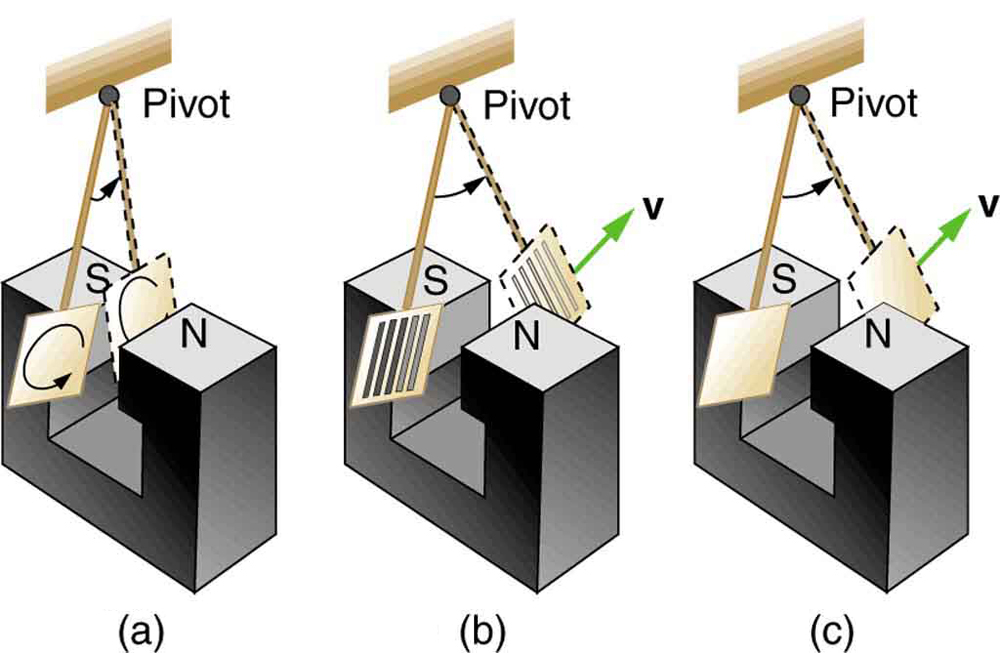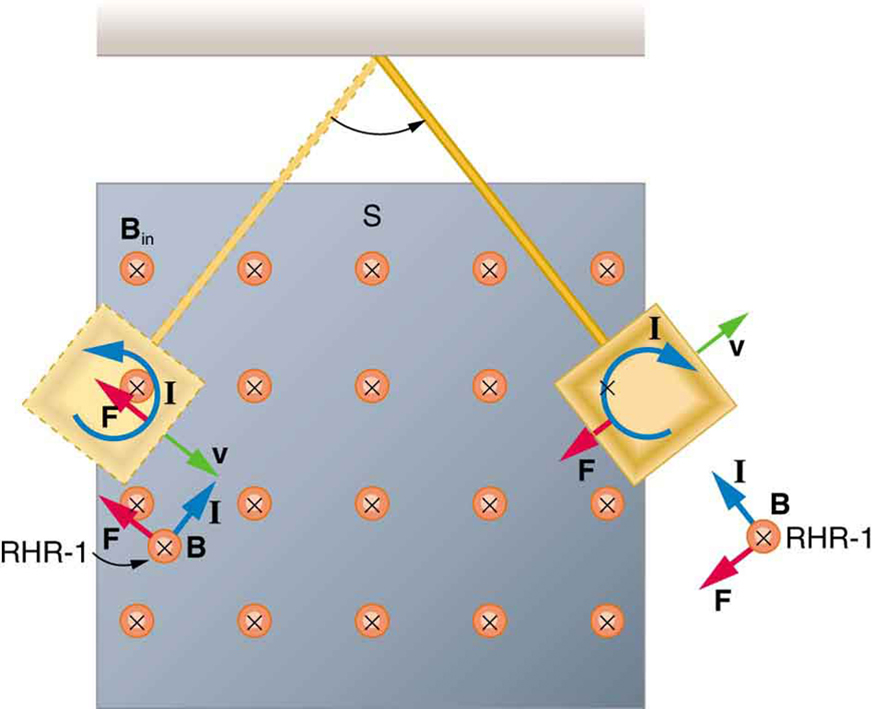# 23.4 Eddy currents and magnetic damping

 Page 1 / 4
• Explain the magnitude and direction of an induced eddy current, and the effect this will have on the object it is induced in.
• Describe several applications of magnetic damping.

## Eddy currents and magnetic damping

As discussed in Motional Emf , motional emf is induced when a conductor moves in a magnetic field or when a magnetic field moves relative to a conductor. If motional emf can cause a current loop in the conductor, we refer to that current as an eddy current    . Eddy currents can produce significant drag, called magnetic damping    , on the motion involved. Consider the apparatus shown in [link] , which swings a pendulum bob between the poles of a strong magnet. (This is another favorite physics lab activity.) If the bob is metal, there is significant drag on the bob as it enters and leaves the field, quickly damping the motion. If, however, the bob is a slotted metal plate, as shown in [link] (b), there is a much smaller effect due to the magnet. There is no discernible effect on a bob made of an insulator. Why is there drag in both directions, and are there any uses for magnetic drag?A common physics demonstration device for exploring eddy currents and magnetic damping. (a) The motion of a metal pendulum bob swinging between the poles of a magnet is quickly damped by the action of eddy currents. (b) There is little effect on the motion of a slotted metal bob, implying that eddy currents are made less effective. (c) There is also no magnetic damping on a nonconducting bob, since the eddy currents are extremely small.

[link] shows what happens to the metal plate as it enters and leaves the magnetic field. In both cases, it experiences a force opposing its motion. As it enters from the left, flux increases, and so an eddy current is set up (Faraday’s law) in the counterclockwise direction (Lenz’s law), as shown. Only the right-hand side of the current loop is in the field, so that there is an unopposed force on it to the left (RHR-1). When the metal plate is completely inside the field, there is no eddy current if the field is uniform, since the flux remains constant in this region. But when the plate leaves the field on the right, flux decreases, causing an eddy current in the clockwise direction that, again, experiences a force to the left, further slowing the motion. A similar analysis of what happens when the plate swings from the right toward the left shows that its motion is also damped when entering and leaving the field.A more detailed look at the conducting plate passing between the poles of a magnet. As it enters and leaves the field, the change in flux produces an eddy current. Magnetic force on the current loop opposes the motion. There is no current and no magnetic drag when the plate is completely inside the uniform field.

When a slotted metal plate enters the field, as shown in [link] , an emf is induced by the change in flux, but it is less effective because the slots limit the size of the current loops. Moreover, adjacent loops have currents in opposite directions, and their effects cancel. When an insulating material is used, the eddy current is extremely small, and so magnetic damping on insulators is negligible. If eddy currents are to be avoided in conductors, then they can be slotted or constructed of thin layers of conducting material separated by insulating sheets.

how do you calculate the 5% uncertainty of 4cm?
4cm/100×5= 0.2cm
haider
how do you calculate the 5% absolute uncertainty of a 200g mass?
= 200g±(5%)10g
haider
use the 10g as the uncertainty?
melia
haider
topic of question?
haider
the relationship between the applied force and the deflection
melia
sorry wrong question i meant the 5% uncertainty of 4cm?
melia
its 0.2 cm or 2mm
haider
thank you
melia
Hello group...
Chioma
hi
haider
well hello there
sean
hi
Noks
the meaning of phrase in physics
is the meaning of phrase in physics
Chovwe
write an expression for a plane progressive wave moving from left to right along x axis and having amplitude 0.02m, frequency of 650Hz and speed if 680ms-¹
how does a model differ from a theory
To use the vocabulary of model theory and meta-logic, a theory is a set of sentences which can be derived from a formal model using some rule of inference (usually just modus ponens). So, for example, Number Theory is the set of sentences true about numbers. But the model is a structure together wit
Jesilda
with an iterpretation.
Jesilda
what is vector quantity
Vector quality have both direction and magnitude, such as Force, displacement, acceleration and etc.
Besmellah
Is the force attractive or repulsive between the hot and neutral lines hung from power poles? Why?
what's electromagnetic induction
electromagnetic induction is a process in which conductor is put in a particular position and magnetic field keeps varying.
Lukman
wow great
Salaudeen
what is mutual induction?
je
mutual induction can be define as the current flowing in one coil that induces a voltage in an adjacent coil.
Johnson
how to undergo polarization
show that a particle moving under the influence of an attractive force mu/y³ towards the axis x. show that if it be projected from the point (0,k) with the component velocities U and V parallel to the axis of x and y, it will not strike the axis of x unless u>v²k² and distance uk²/√u-vk as origin
show that a particle moving under the influence of an attractive force mu/y^3 towards the axis x. show that if it be projected from the point (0,k) with the component velocities U and V parallel to the axis of x and y, it will not strike the axis of x unless u>v^2k^2 and distance uk^2/√u-k as origin
No idea.... Are you even sure this question exist?
Mavis
I can't even understand the question
yes it was an assignment question "^"represent raise to power pls
Gabriel
Gabriel
An engineer builds two simple pendula. Both are suspended from small wires secured to the ceiling of a room. Each pendulum hovers 2 cm above the floor. Pendulum 1 has a bob with a mass of 10kg . Pendulum 2 has a bob with a mass of 100 kg . Describe how the motion of the pendula will differ if the bobs are both displaced by 12º .
no ideas
Augstine
if u at an angle of 12 degrees their period will be same so as their velocity, that means they both move simultaneously since both both hovers at same length meaning they have the same length
Modern cars are made of materials that make them collapsible upon collision. Explain using physics concept (Force and impulse), how these car designs help with the safety of passengers.
calculate the force due to surface tension required to support a column liquid in a capillary tube 5mm. If the capillary tube is dipped into a beaker of water
find the time required for a train Half a Kilometre long to cross a bridge almost kilometre long racing at 100km/h
method of polarization
Ajayi
What is atomic number?
The number of protons in the nucleus of an atom
Deborah

#### Get Jobilize Job Search Mobile App in your pocket Now!ByBy OpenStaxBy John GabrieliBy David CoreyBy Brooke DelaneyBy Alec MoffitBy Brooke DelaneyBy Madison ChristianBy MldelatteBy Subramanian DivyaBy JavaChamp Team